﻿ G Super-Complex Space-Time TheoryPublications are Open
Access in this journal
Article Versions
Export Article
• Normal Style
• MLA Style
• APA Style
• Chicago Style
Original Article
Open Access Peer-reviewed

### G Super-Complex Space-Time Theory

Guan Yiying , Guan Tianyu, Yang Fan, Dai Qing, Zhang Yang
International Journal of Physics. 2018, 6(1), 18-25. DOI: 10.12691/ijp-6-1-4
Published online: January 22, 2018

### Abstract

The real and imaginary parts of complex physical quantities reflect the mutual contradictory relationship within the unity, including the unity and opposition, correlation dependence and mutual transformation. The quarks, leptons, fermions and bosons are same particles at different kinestate in complex space-time. The velocity vector generated by the rotation of the particles in the complex space distracts the time dimension, the vector forms the weak charge in the space projection; its rotary angular velocity vector distracts the energy dimension, the vector forms the mass charge in the space projection; Its rotary centripetal acceleration vector distracts the space dimension to form the electric charge; the acceleration jerk (variation of acceleration) generated by the rotation of the vector distracts the color dimension, the vector forms the color charge in the space projection; the projections of the electric charge, mass charge, color charge and weak charge into the 3D space form the three-nature (positive, negative and neutral electrodes), three-generation, three-boson and the past, present and future of particles; the empty space is a zero rest mass, linear and superposed, non-dispersive, holographic and open string boson state; the charge, rest mass and gravity are all changed with the temperature and kinematic velocity of particles. The universe is holographic, bounded and boundless. All information within the universe can be saved in the form of holographic image produced by the wave interference onto the horizon-surface, where the substances establish universal relation with those (mainly as the fermion state) in the universe by means of quantum entanglement.

### 1. Introduction

The French Suke obtained an imaginary root in the Three Chapters on Arithmetic when solving the quadratic equation in 1484. 61 years later, Kadel carefully introduced the imaginary number in solving the quadratic equation. It is until 1637 that Descartes first defined the term “imaginary” in the Geometry as response to “real”. Euler first uses i as an imaginary unit in the paper titled Formula of Differentiation in 1777. Agung from Geneva came up with the concepts of “model” and argument and the triangle expression of complex numbers in 1806. Minkowski introduced the four-dimensional space-time and the time coordinate of imaginary number x4=ict in 1908. Schrodinger described the status of microscopic particle with the wave function (complex variables function) in quantum theory in 1926; the (a real value) of Hermitian operator in response to mechanical quantity was used as the supposed value of observable; it was thus the Schrodinger wave equation was established. The quantum mechanics was really set up from that time on 1. Why is Relativity questioned since its establishment while the theory of quantum physics and alternating currents advances by rushes and turns to a well-established theory? The reason is that imaginary number is introduced in the theory of quantum physics and alternating currents. However, the imaginary number in such fields is introduced as a mathematical tool which facilitates computation and without interpretation of its physical significance. In fact, the physical significance of imaginary number is that it reflects the existence of a reverse system in both opposition to and unity with the real system.

### 2. Complex Expression of Physical Parameters in G complex Space-time

2.1. Velocity Expression

When θ = ωt is put in formula (2.1.1) to derive t, the complex velocity will be: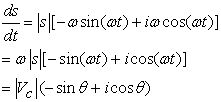(2.1.1)

That is,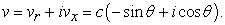(2.1.2)

The expression of space-time angle obtained is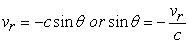(2.1.3)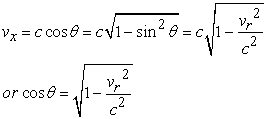(2.1.4)

(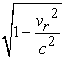is Lorentz coefficient of expansion).

Demonstration is given below with the geometry method:

As shown in Figure.1, move the velocity vector C of particle at point Q horizontally to the origin, (the velocity vector is horizontally moved to the origin. y is the time axis, and y =ict. x is the space axis. c is the velocity of light)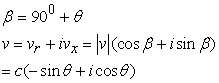(2.1.5)

End.

• Figure 1. G complex space-time velocity
2.2. Expression of Acceleration in G complex Space-time

By using the formula (2.1.1), the complex acceleration is obtained by taking two derivatives of t: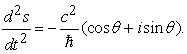(2.2.1)

Demonstration is given below with the geometry method:

• Figure 2. G complex space-time acceleration

As shown in Figure 2, move the acceleration vector of particle at point Q to the origin to get a,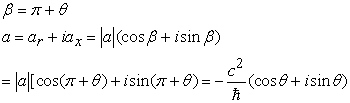(2.2.2)

Assume that the real part equals to the imaginary part for: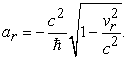(2.2.3)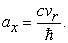(2.2.4)

So the algebraic expression of acceleration in G complex space-time is: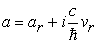(2.2.5)

When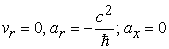will be obtained

When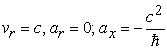will be obtained.

It indicates that any object moving at the velocity of light cannot be accelerated in terms of the real space-time.

2.3. Expression of G Complex Space-time

According to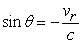when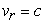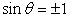will be obtained. In this case,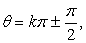which means that when the space H moves at the velocity of light, the projection of the space length on the static space S will be zero. That is to say that the space H is purely imaginary space (pure time) and perpendicular to the static space (purely real space) S. So, The complex space-time can be written as the direct-sum space of space and time, that is,: The space-time complex number of particle at point Q can be written in the formula below: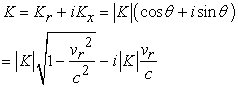(2.3.1)

(Kr is the three-dimensional space with the attribute of Riemann's non-Euclidean geometry; Kx is the one-dimensional imaginary space (time) with the attribute of Lobachevskian geometry.)

If the three-dimensional space Kr is contracted to the one-dimensional x, and assume that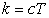(2.3.2)

(c is the velocity of photon in empty space; T is the empty space-time; the velocity (of the matter of which the rest mass is not zero or of photon in the medium) is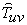(or the projection of velocity of light on space). The time is t that is called dielectric or ordinary time). The relationship is: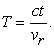(2.3.3)

Substituting (2.3.2) for (2.3.3) above in (2.3.1) to get: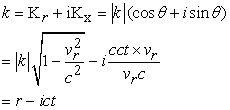(2.3.4)

(c is the velocity of light; r is the spatial distance; t is dielectric or ordinary time)

The formula above serves as the mathematical basis of complex space-time planimetry.

According to the complex number theorem,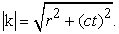(2.3.5)

It can be said that the quantum probability is caused by the introduction of the imaginary number i into the Schrodinger equation, that is, Schrodinger introduced into his equation the virtual space (i.e. time) which is opposite and unified with the spatial entity. Because all particles are moving in complex spacetime, we cannot observe the particles when they are moving in the void (time), but when the wave function is squared, the imaginary part of the particle is eliminated, so. Only the square of wave function is the physical quantity we can measure. It is only the essence of quantum probability.

### 3. The Relationship between Physical Quantities

3.1. Relationship between Magnetic Charge and Electric Charge

It is believed in the theory of G complex space-time that: complex electric charge is the centripetal acceleration of the matter taking circular motion in the complex space-time. Its projection in the real space is developed into the electrostatic charge. A charged particle is assumed at point q in the complex space-time. Its direction of centripetal acceleration is (as shown in Figure 2).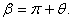Expression with the projection operator: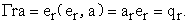(3.1.1)

The complex charge is assumed to be: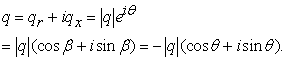(3.1.2)

Assume that the real and the imaginary parts in the formula above are the same: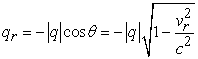(3.1.3)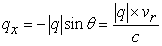(3.1.4)

(qr is the projection of complex charge on the real space or called electrostatic charge (sometimes called “charge” for short).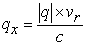is the projection of complex charge on the time line and called magnetic charge). Electric charges develop the electrostatic field where electrons achieve linear motion; magnetic charges (or the moving electric charges) develop the magnetic field where the moving electrons achieve spiral motion. The interaction of electric and magnetic fields produces electromagnetic wave.

When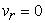, we get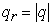and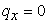When, we get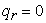and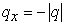.

When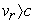,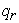will be an imaginary number. According to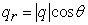, when the space-time angle exceeds 90 degrees, a negative cosine value will be obtained. The imaginary number is a symbol of the reverse system. In other words, when velocity of the object turns to zero, the maximum real charge (static charge) of the particle will be achieved and equals to the total charges. When the object moves at greater velocity, the electrostatic charges will be reduced and imaginary charges will be increased; however, the model of charge is a constant. When velocity of the object equals to that of light, electrostatic charges of the particle will turn to zero. When it moves at the rate greater than the velocity of light, original electrostatic charges will exist in the form of antielectron, i.e. positive electron.

3.2. Electric Charge and Temperature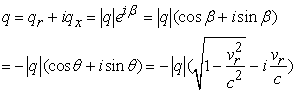(3.2.1)

(3.2.1) type of real imaginary part is equal to:(3.2.2)(3.2.3)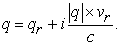(3.2.4)

According to the thermodynamics theory, the relation between the average rate of the ideal gas molecule and the temperature in the equilibrium state is as follows 1: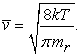(3.2.5)

The T is the temperature,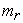the static mass, the K as the Boltzmann constant (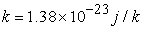).

The upper into (3.2.1) form is: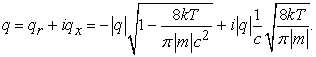(3.2.6)

In which q is a charge.

The absolute value of the real charge decreases as the T rises, and the absolute value of the Coulomb force decreases, and the absolute value of the magnetic charge will increase; and vice versa.

### 4. Super-complex Space-time Model

4.1. G Complex Space-time 2D Model

The cross product (“rotation”) of space vector generates time perpendicular to it; and the cross product of time and space generates energy perpendicular to space-time. 3D space with superluminal motion generates a 1D virtual space also called time axis T(ic) perpendicular to motionless space(R), the particle G will do uniform circular motion in space R and time T (“virtual specific). The time and space of any particle can be measured in complex space-time. Time and space are unity of opposites and mutual transformation. The anticlockwise rotation angle speed in complex space-time generates positive energy (“mass”) particle in vertical direction (conform to “right-hand screw rule”), conversely clockwise rotation generates negative energy particle. The centripetal acceleration occurring in rotation generates electric charge in space-time, its projection on real space generates positive and negative static electric charge, the projection on time axis generates magnetic charge. The particle rotation can be regarded as a matter doing circumferential uniform motion along 0-D-F-B, showing positive charge on D-F-B period and negative on B-O-C period. Particle rotation is equal to space-time rotation. Magnetic charge relates to electric charge while G charge relates to mass charge. The magnetic charge electric charge can be mutual transformation as well as G charge and mass charge. The projection of above process on space-time axis is similar to a vibration process of two harmonic vibrators perpendicular to each other, in other words, the particle vibrating composition of time and space that perpendicular to each other will make the matter do circumferential uniform motion in complex space-time. The vibrator vibration will produce a circle matter wave (as shown in Figure 3) perpendicular to space-time level. The matter motion is un-continuous and dispersed, means the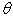angle cannot take continue value.

• Figure 3. The two-dimensional model of G complex space-time

The above process is also equal to a pair of positive and negative electrons generated at D the negative electron moving in the direction of D-F-B, the positron electron moving in the direction of D-O-B, they annihilate at point B.

4.2. G Complex Space-time Ring Model

We call all kinds of rotation and motion methods of particle G in 2D space and 1D virtual space as particle G 3D model (as shown in Figure 4). Wherein, AB-O-CD area (i.e. “double horn area”) is state material area with negative time, negative space, negative energy and negative curvet which is conform to Roche Geometric Properties; while AB- FE-CD area (i.e. ball outside surface) is normal material state area with positive space, positive energy, positive time direction and positive curvet which is conform to Riemannian Geometric Properties. AB, DC constitute horizon ring.

4.3. G Complex Space-time Quark Model
4.3.1. Quarks Angle of Space-time

In complex space-time coordinate plane, the angle between matter vector and space coordinates is defined as space-time angle of the vector (as shown in Figure .5). Assume the quark angle isthe ant quarks compound angle calculation formula is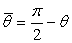.

• Figure 4. The three-dimensional model of G complex space-time
• Figure 5. Quark model

The space-time angle of up quark u, charm quark c and top quark t is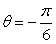with the antiquary angle of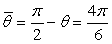; the space-time angle of down quark d, strange quark s and bottom quark b is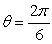with the antiquary angle of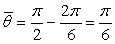; the space-time angle of gluon is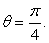We can safely see that: the three dimensions perpendicular to each other in real space have same space-time angle, in other words, quark u, quark c and quark t are projection vectors on three coordinates perpendicular to each other in real space by particle G with the space-time angle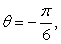which also explained how quark and three lepton generations came from; quark d, quark s and quark b are projection vectors on 3D real space coordinates by particle G with the space-time angle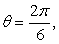the rotation of particle G in complex space-time is equal to rotation of open quark in 3D real space (“Accelerated motion”). Quark u, quark c and quark t will transferred them into quark d, quark s and quark b in the accelerated motion in 3D real space and became virtual particle to each other since they have a space-time angle difference of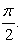4.3.2. Charge Value of Quark

The negative value on real part of particle centripetal acceleration in complex space-time is defined as electric charge, namely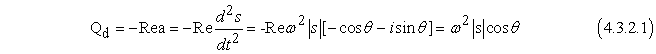make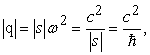then: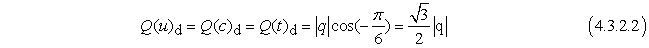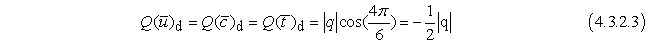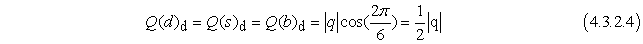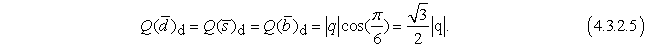4.3.3. Color Properties of Quarks

4.3.3.1. Color charge particle in complex jerk time (rotation acceleration changes) opened its projection in the space noted that, forming a color charge.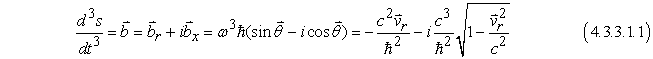real part of the color charge is: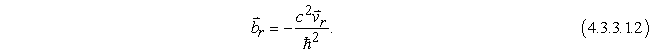The absolute value of the color charge increases with the increase of velocity. It shows that the color force plays a major role in the early or microcosmic fields of the universe.

4.3.3.2. Three colors

The projection of the color vector in three-dimensional space forms three colors.

Quaternion of color force is expressed as so there is 2: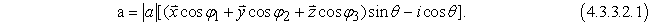4.3.3.3. Color force

Definition of color force: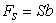"b" signifies for jerk whilst "S" signifies quantity correlating to color force. "S" is likely to be mass (hereafter hypothesized as mass "m").

Color force: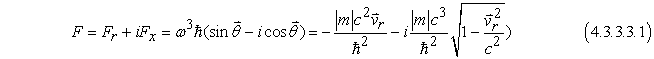So, after expanding to quaternion, formula (4.3.3.3.1) can be represented as: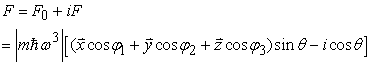OrAnd there are: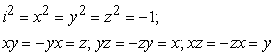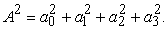The real part of (4.3.3.3.2) is: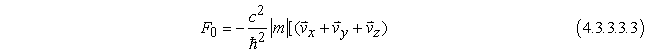in which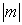is the module value of mass.

The aforementioned formula indicates:

1. Color force of real part positively is in direct proportion to product between particle energy and its kinematic velocity. The greater the energy or velocity, the greater the absolute value of color force. As the particles in the early universe or in the microcosmic world are in a state of high energy and high-velocity motion, the intensity of real part color force is much greater than other forces.

2. Color force is in the opposite vector to particle velocity, this feature determines that the quark is closely confined.

3. When the two quarks shot close to each other, the relative velocity between them gradually reduces, so the color force also gradually reduces. This reveals the reason for the asymptomatic freedom of quarks.

4. The space angle of quark is greater than zero yet less than 90 degrees, so quark carries fractional charge and color charge.

5. As the typical fermions are located in the space, meantime with space-time angle being zero, the real part color force (color charge) of typical fermions (such as electronics, μ particle and τ particle) is zero, hence not involved in strong interaction.

The above formula shows that the absolute value of imaginary part color force decreases as the velocity increases.

The above formula signifies: The projection of color charge in 3-D space can form three-color charges (red, blue, green). The color space does not alter the space-time angle (i.e. the conformal). So the color effect (strong interaction) does not alter the electrostatic charge or static mass of the particles and it has the following attributes:

Any quark has three colors. This is because, each quark vector has three angles with three dimensions in space (e.g.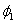,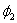,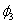), and the square of the cosine of its angle constitutes a property of "color" of quark. So,

4.3.4. Quark Weak Force and Weak Charge

Weak charge: The velocity vector of a particle in a complex spacetime opens up the time dimension. Its projection in space forms a weak charge.

That is: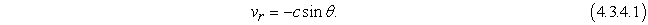The weak force is defined as the real part of the complex momentum of the particle (projecting in the space), forming the weak force.

That is: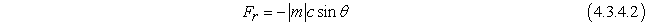where v is the velocity. P is momentum.

So the complex form of weak force in g complex spacetime (four momentum form) is: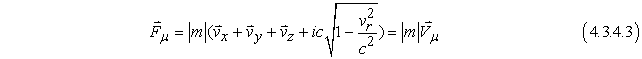(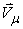is four speed,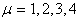) to show that the real weak force is proportional to the momentum of the particle. The larger the momentum, the stronger the weak force and the easier it is to decay. The weak force is also related to the space-time angle (particle velocity). The greater the space-time angle (velocity), the stronger the weak force, so the easier it is to decay. The mass of neutrons is slightly larger than the mass of protons. Moreover, the neutron's space-time angle is 90 degrees, which is larger than the proton's space-time angle of 0 degree, so the neutron decays more easily than the proton.

4.3.5. The Mass Force and Mass Charge of Quarks (Newton Force)

Energy: The angular velocity of the circular motion of the particle in the complex space-time opens up the energy dimension.

Complex energy formula: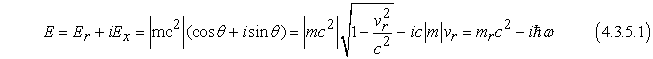(The first item on the right is Einstein's equation of mass-energy, The second term is Planck's energy equation 3).

According to the complex number theorem: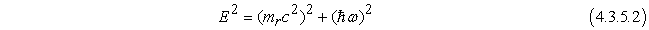Mass force: Energy’s change(gradient)in space forms mass force.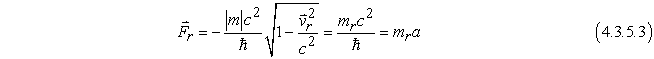Mass charge: The real part of the energy (the projection of the space) is divided by the square of the speed of light.

That is: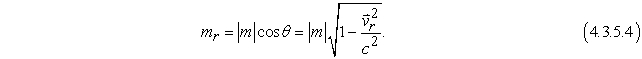The velocity vector generated by the rotation of the particles in the complex space distracts the time dimension, the vector forms the weak charge in the space projection; its rotary angular velocity vector distracts the energy dimension, the vector forms the mass charge in the space projection; Its rotary centripetal acceleration vector distracts the space dimension to form the electric charge; the acceleration jerk (variation of acceleration) generated by the rotation of the vector distracts the color dimension, the vector forms the color charge in the space projection; the projections of the electric charge, mass charge, color charge and weak charge into the 3D space form the three-nature (positive, negative and neutral electrodes), three-generation, three-color and the past, present and future of particles.

### 5. G Vacuum Model

• Definition of vacuum: state of matter with rest mass of 0.

• In vacuum theorem 1, matter exists in form of wave.

• In vacuum theorem 2 Matters in vacuum are linear.

• In vacuum theorem 3 Matters in vacuum can be superposed.

• In vacuum theorem 11Matters are non-dispersive (dispersity is o)

• In vacuum theorem 4 All matters in vacuum move at velocity of light.

### 6. G Complex Space-time Cosmological Model

6.1. Non-Euclidean Universe

Set the cosmological function as: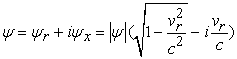(6.1.1)

Where the static characteristics of material are reflected in the real part, forming the Riemann space 4; the dynamic characteristics of material represented in the imaginary part, forming the Roche space 5.

In the three-dimensional space, the universe seems like a photosphere whose glossy is described as visual interface. Together, the visual interface of universe is equipotential surface as well, with the rest mass and gravitation being zero. The visual interface is comprised by wave-like material that is linear, stackable as well as non-dispersive. In other word, visual interface is considered as Boson Ocean. The above characteristics are fully embodied in the imaginary part of cosmological function. The inside content of visual interface is called as Fermion Ocean with involvement of rest mass and gravitation. Therefore, cosmological function has achieved all the characteristics above by following the features of non-linear, non-stackable and dispersive.

6.2. Bounded Immense Universe

Matter moving at velocity of light consists of interface of positive and negative universe. Observers in the interface can still think that the universe they live in is infinite, because, all rulers of observers approaching interface continuously are continuously shortened with the continuous increasing of velocity according to formula of relativity theory. Hence, the observers can measure infinitely with their rulers which will never come to border of universe comprising of matters moving at velocity of light and this is the unity of being finite and infinite.

Through the white hole, anti-universe is the source of positive universe, whereas positive universe is the source of anti-universe through the black hole. The concept is similar to the Chinese diagram of universe constituted by yin and yang of fish. Furthermore, the chirality of positive universe and anti-universe is opposite.

6.3. Oscillatory Universe

will form after projection of Figure 4 into two-dimensional space.

The early universe expands increasingly at super velocity of light and is repulsive force-based and Lobachevskian space, with negative space curvature and is a negative energy state area; when universe expands to certain dimension (r=rc or t=tc), the universe begins to slow down expansion at low velocity of light and is attractive force-base and Riemann space, with positive space curvature and is a positive energy state area. With gravitational action when universe expands to certain degree, it begins to accelerate shrink and it is Riemann space still in which is mainly attractive force still; when it shrinks to certain dimension (r=rc or t=tc, i.e. horizon surface), the universe begins to slow down shrink at super velocity of light and at the time it is repulsive force-bases and is Lobachevskian space again. The universe moves in circles in that way.

6.4. Holographic

And then form a visual interface of universe (suction surface) by the luminal motion material. With the interference of linear waves, the hologram is obtained (so that every point on the visual interface contains the information of the entire glossy and universe).

6.5. Universally Relationship

The entanglement of two particles is through the phase (imaginary part) of two particles. It can also be said that the entanglement of particles is realized on the visual interface (positive and negative cosmic interface), while the virtual particles (field particles) are excited to form real particles. The real particle and the field particle satisfy the relation between the real part and the imaginary part of the complex number, and the real imaginary part satisfies the Cauchy Riemann equation.

6.6. Multiple Universes

When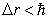(it can be in analogy with Shivas radius)) or △t<△tc; i.e. if activity space of space is small enough or existence time of matter is short enough, then its inner space, mass and electric charge will change into reverse signs, namely change into reverse system of their own, that is to say: The original real space changes into negative space. In other words, at the time space-time turns into anti space-time (microcosm) and matter turns into antimatter; there is horizon surface consisting of velocity of light between microcosm and our macrocosm and there special areas (microcosm) will generate a large number of small black holes and white holes in our present macrocosm.

### 7. Conclusion

G complex space-time theory has described the entire universe in simper words. It has deeply revealed the nature of time and space, real space and imaginary space, continuous and discrete, finite and infinite, normal and inverse state, particle and wave of the material. Although G complex space-time theory needs to be developed and perfected, it is no doubt that it truly reflects the beauty of harmony and simper in nature. It is a rising star, and it will become a bright pearl of scientific palace in the future.

### References

  Shouzhu River forever general physics in Higher Education Press Beijing 1982 p264. In article  Yang Piwen four element analysis and partial differential equations in Science Press Beijing 2009 P2. In article  Gordon Fraser Qin Kecheng twenty-first Century New Physics Science Press Beijing 2013 p99. In article  Chen Weiheng Li Xing Riemann school P83. In article  Peking University Press 2002 geometric introduction to Russian LObachevsky Kutuzov LObachevsky geometry and geometric basic overview Harbin Institute of Technology press 2012 p57. In article

Published with license by Science and Education Publishing, Copyright © 2018 Guan Yiying, Guan Tianyu, Yang Fan, Dai Qing and Zhang YangThis work is licensed under a Creative Commons Attribution 4.0 International License. To view a copy of this license, visit http://creativecommons.org/licenses/by/4.0/